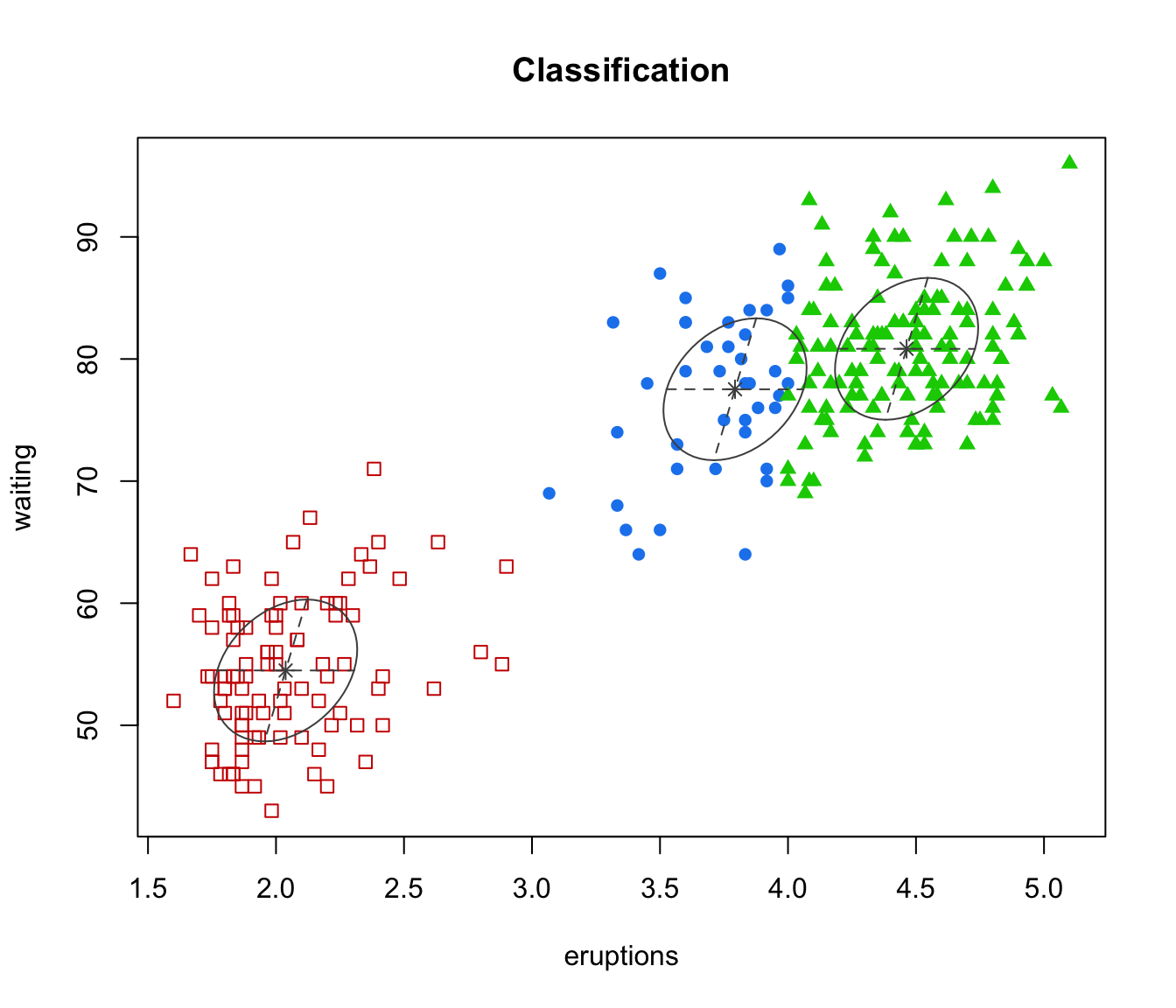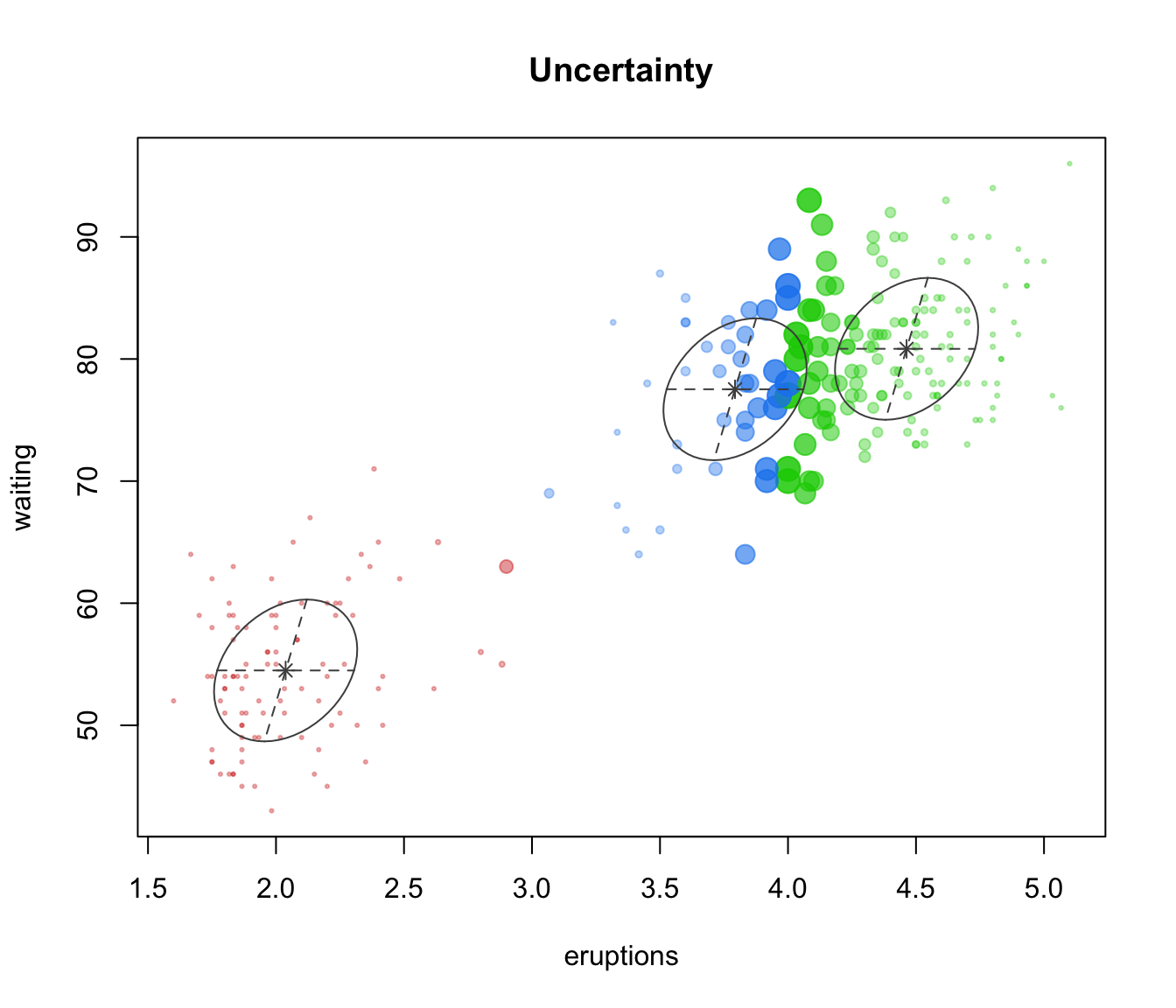Plot two-dimensional data given parameters of an MVN mixture model for the data.

## Usage

mclust2Dplot(data, parameters = NULL, z = NULL,
classification = NULL, truth = NULL, uncertainty = NULL,
what = c("classification", "uncertainty", "error"),
addEllipses = TRUE, fillEllipses = mclust.options("fillEllipses"),
symbols = NULL, colors = NULL,
xlim = NULL, ylim = NULL, xlab = NULL, ylab = NULL,
scale = FALSE, cex  = 1, PCH = ".",
main = FALSE, swapAxes = FALSE, ...)

## Arguments

data

A numeric matrix or data frame of observations. Categorical variables are not allowed. If a matrix or data frame, rows correspond to observations and columns correspond to variables. In this case the data are two dimensional, so there are two columns.

parameters

A named list giving the parameters of an MCLUST model, used to produce superimposing ellipses on the plot. The relevant components are as follows:

pro

Mixing proportions for the components of the mixture. There should one more mixing proportion than the number of Gaussian components if the mixture model includes a Poisson noise term.

mean

The mean for each component. If there is more than one component, this is a matrix whose kth column is the mean of the kth component of the mixture model.

variance

A list of variance parameters for the model. The components of this list depend on the model specification. See the help file for mclustVariance for details.

z

A matrix in which the [i,k]th entry gives the probability of observation i belonging to the kth class. Used to compute classification and uncertainty if those arguments aren't available.

classification

A numeric or character vector representing a classification of observations (rows) of data. If present argument z will be ignored.

truth

A numeric or character vector giving a known classification of each data point. If classification or z is also present, this is used for displaying classification errors.

uncertainty

A numeric vector of values in (0,1) giving the uncertainty of each data point. If present argument z will be ignored.

what

Choose from one of the following three options: "classification" (default), "error", "uncertainty".

A logical indicating whether or not to add ellipses with axes corresponding to the within-cluster covariances.

fillEllipses

A logical specifying whether or not to fill ellipses with transparent colors when addEllipses = TRUE.

symbols

Either an integer or character vector assigning a plotting symbol to each unique class in classification. Elements in colors correspond to classes in order of appearance in the sequence of observations (the order used by the function unique). The default is given by mclust.options("classPlotSymbols").

colors

Either an integer or character vector assigning a color to each unique class in classification. Elements in colors correspond to classes in order of appearance in the sequence of observations (the order used by the function unique). The default is given is mclust.options("classPlotColors").

xlim, ylim

Optional argument specifying bounds for the ordinate, abscissa of the plot. This may be useful for when comparing plots.

xlab, ylab

Optional argument specifying labels for the x-axis and y-axis.

scale

A logical variable indicating whether or not the two chosen dimensions should be plotted on the same scale, and thus preserve the shape of the distribution. Default: scale=FALSE

cex

An argument specifying the size of the plotting symbols. The default value is 1.

PCH

An argument specifying the symbol to be used when a classificatiion has not been specified for the data. The default value is a small dot ".".

main

A logical variable or NULL indicating whether or not to add a title to the plot identifying the dimensions used.

swapAxes

A logical variable indicating whether or not the axes should be swapped for the plot.

...

Other graphics parameters.

## Value

A plot showing the data, together with the location of the mixture components, classification, uncertainty, and/or classification errors.

surfacePlot, clPairs, coordProj, mclust.options

## Examples

# \donttest{
faithfulModel <- Mclust(faithful)

mclust2Dplot(faithful, parameters=faithfulModel$parameters, z=faithfulModel$z, what = "classification", main = TRUE)mclust2Dplot(faithful, parameters=faithfulModel$parameters, z=faithfulModel$z, what = "uncertainty", main = TRUE)# }